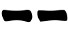## Saturday, 2 July 2016

This method was devised and published on the internet by i@no-self in 2001. I took the liberty of changing the procedure a little to make it easier to memorize.

In this method, an hexagram is selected at random and the line is derived on the basis of the upper and lower trigrams:

1. Open an I Ching book to a random page and look at the hexagram that page relates to;
2. If the lower trigram has one or three yin (broken) lines, you got a yin line:
• if the upper trigram has three broken line, draw;
• otherwise  draw;
3. If the lower trigram has one or three yang (solid) lines, you got a yang line:
• if the upper trigram has exactly one solid line, draw;
• otherwise draw;
4. Repeat steps 1-2 other five times drawing the hexagram from bottom to top.
If you find difficult to memorize the rules, you can use the table below which is structured according the original formulation by i@no-self.

#### Probabilities

The actual probabilities of each line depends on how the book has been typeset (some hexagram may have more pages than others) and by how much one is inclined to pick up the pages that are towards the end or the beginning of the book.
Assuming, everything considered, that any combination of upper/lower trigrams has the same probability to be selected, the probabilities for each line are the same as the Method of 16:

Prob(6) = 1/16
Prob(8) = 7/16
Prob(7) = 5/16
Prob(9) = 3/16
Prob(yin) = Prob(yang) = 1/2